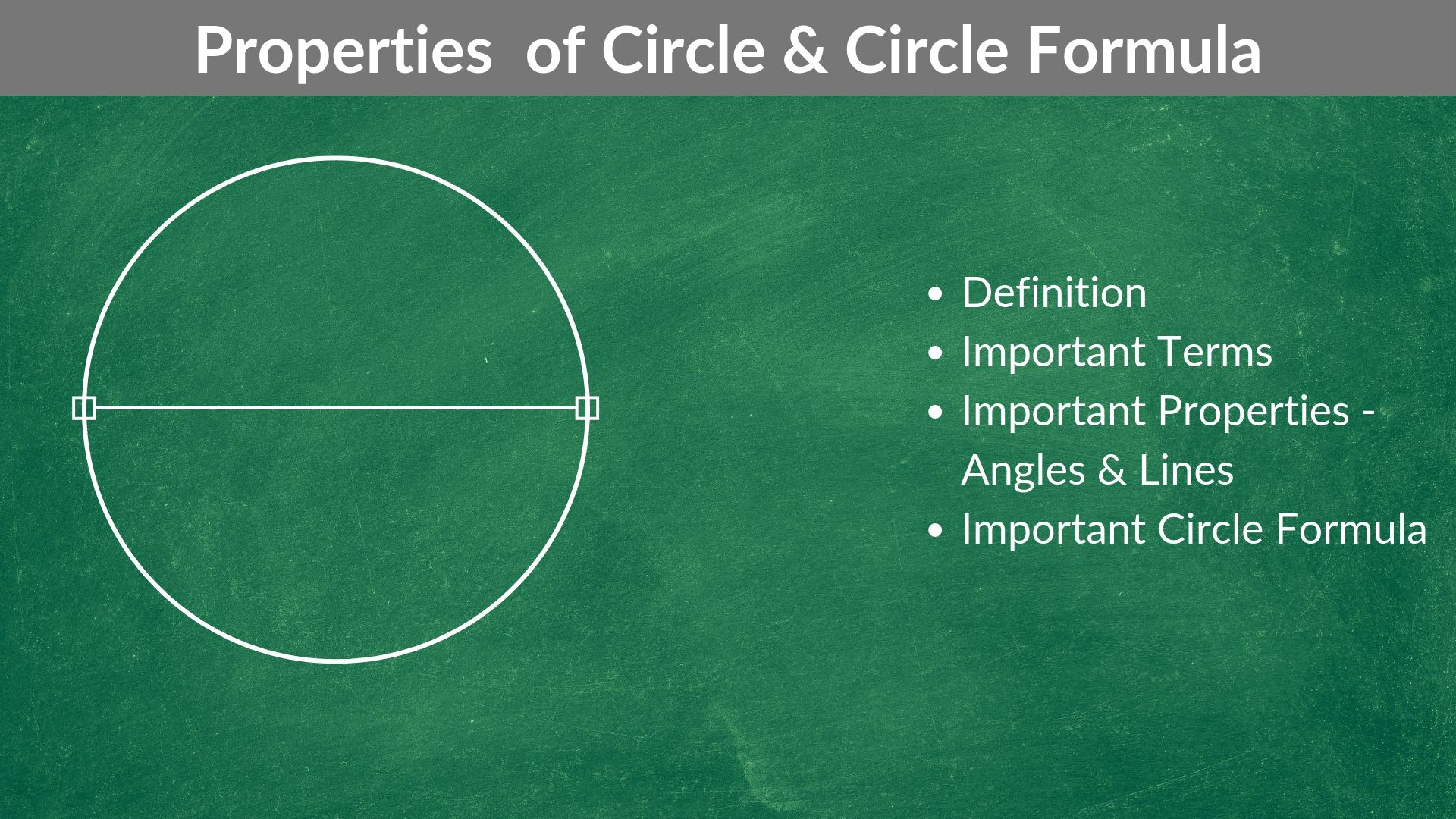# What is the greatest number of blocks 8 centimetres by 6 centimetres by 9 centimetres that will fit into a storage space that is 60 centimetres by 72 centimetres by 96 centimetres? – OGQR 2020 Question #101 with Solution

OGQR 2020: Question No. 101 What is the greatest number of blocks 8 centimetres by 6 centimetres by 9 centimetres that will fit into a storage space that is 60 centimetres by 72 centimetres by 96 centimetres? Source OGQR 2020 Type Problem Solving Topic Geometry Sub-Topic Rectangular Solid Difficulty Easy – Medium Solution Given In

Read More# In the figure above, lines are drawn at the vertices of the quadrilateral as shown. What is the sum of the degree measures of the marked angles? – OGQR 2020 Question #92 with Solution

OGQR 2020: Question No. 92 In the figure above, lines are drawn at the vertices of the quadrilateral as shown. What is the sum of the degree measures of the marked angles? Source OGQR 2020 Type Problem Solving Topic Geometry Sub-Topic Quadrilateral Difficulty Medium Solution Given In this question, we are given The figure of

Read More# In the figure above, RST is a triangle with angle measures as shown and PRTQ is a line segment. – OG 2020 Question #306 with Solution

OG 2020: Question No. 306 In the figure above, RST is a triangle with angle measures as shown and PRTQ is a line segment. What is the value of x + y? s = 40 r = 70 Source OG 2020 Type Data Sufficiency Topic Geometry Sub-Topic Triangles Difficulty Easy – Medium Solution Steps 1

Read More# In the figure above, if triangles ABC, ACD, and ADE are isosceles right triangles and the area of ΔABC is 6, then the area of ΔADE is – OGQR 2020 Question #87 with Solution

OGQR 2020: Question No. 87 In the figure above, if triangles ABC, ACD, and ADE are isosceles right triangles and the area of ΔABC is 6, then the area of ΔADE is: Source OGQR 2020 Type Problem Solving Topic Geometry Sub-Topic Triangle Difficulty Medium Solution Given In this question, we are given A diagram consists

Read More# If a rectangle of area 24 can be partitioned into exactly 3 nonoverlapping squares of equal area, what is the length of the longest side of the rectangle? – OGQR 2020 Question #84 with Solution

OGQR 2020: Question No. 84 If a rectangle of area 24 can be partitioned into exactly 3 nonoverlapping squares of equal area, what is the length of the longest side of the rectangle? Source OGQR 2020 Type Problem Solving Topic Geometry Sub-Topic Quadrilateral Difficulty Medium – Hard Solution Given In this question, we are given

Read More# The figure above shows a piece of cheese with a corner cut off to expose plane surface ABC. What is the area of surface ABC? – OGQR 2020 Question #221 with Solution

OGQR 2020: Question No. 221 The figure above shows a piece of cheese with a corner cut off to expose plane surface ABC. What is the area of surface ABC? AD = 10 centimetres The shape of the cheese was a cube before the corner was cut off Source OGQR 2020 Type Data Sufficiency Topic Geometry Sub-Topic Cubes (3D)/Triangles Difficulty

Read More# In the racetrack shown above, regions I and III are semi-circular with radius r. If region II is rectangular and its length is twice its width, what is the perimeter of the track in terms of r? – OGQR 2020 Question #94 with Solution

OGQR 2020: Question No. 94 In the racetrack shown above, regions I and III are semi-circular with radius r. If region II is rectangular and its length is twice its width, what is the perimeter of the track in terms of r? Source OGQR 2020 Type Problem Solving Topic Geometry Sub-Topic Circle / Rectangle Difficulty

Read More# When a rectangular vat that is 3 feet deep is filled to (2/3) of its capacity, it contains 60 gallons of water. If 7 1/2 gallons of water occupies 1 cubic foot of space, what is the area, in square feet, of the base of the vat? – OGQR 2020 Question #96 with Solution

OGQR 2020: Question No. 96 When a rectangular vat that is 3 feet deep is filled to (2/3) of its capacity, it contains 60 gallons of water. If 7 1/2 gallons of water occupies 1 cubic foot of space, what is the area, in square feet, of the base of the vat? Source OGQR 2020

Read More# If the diameter of a circular skating rink is 60 meters, the area of the rink is approximately how many square meters? – OGQR 2020 Question #100 with Solution

Read MoreRead More